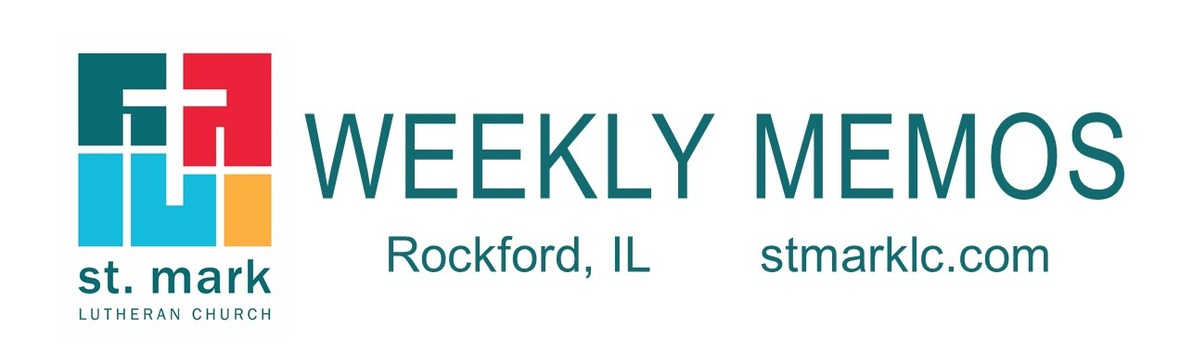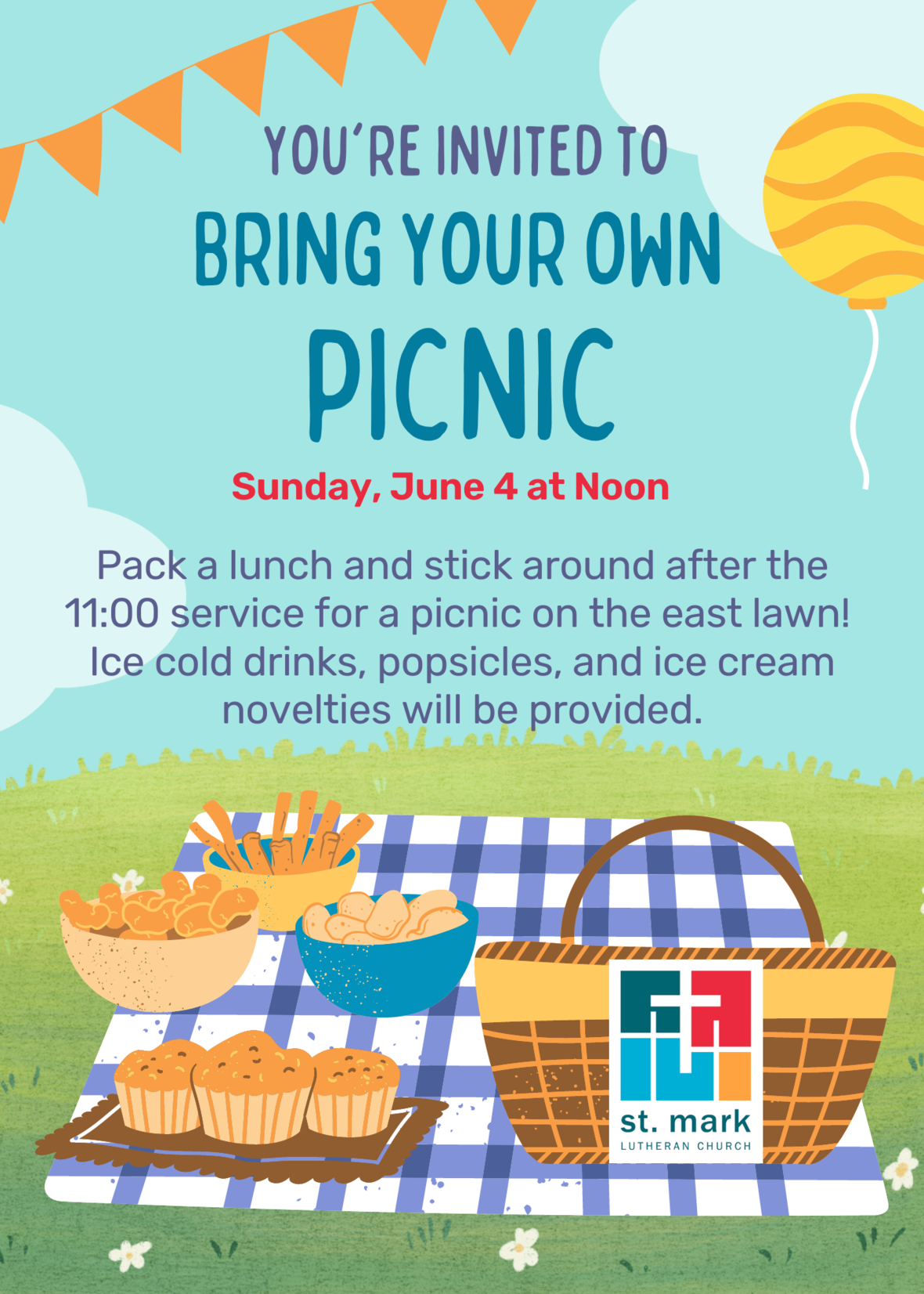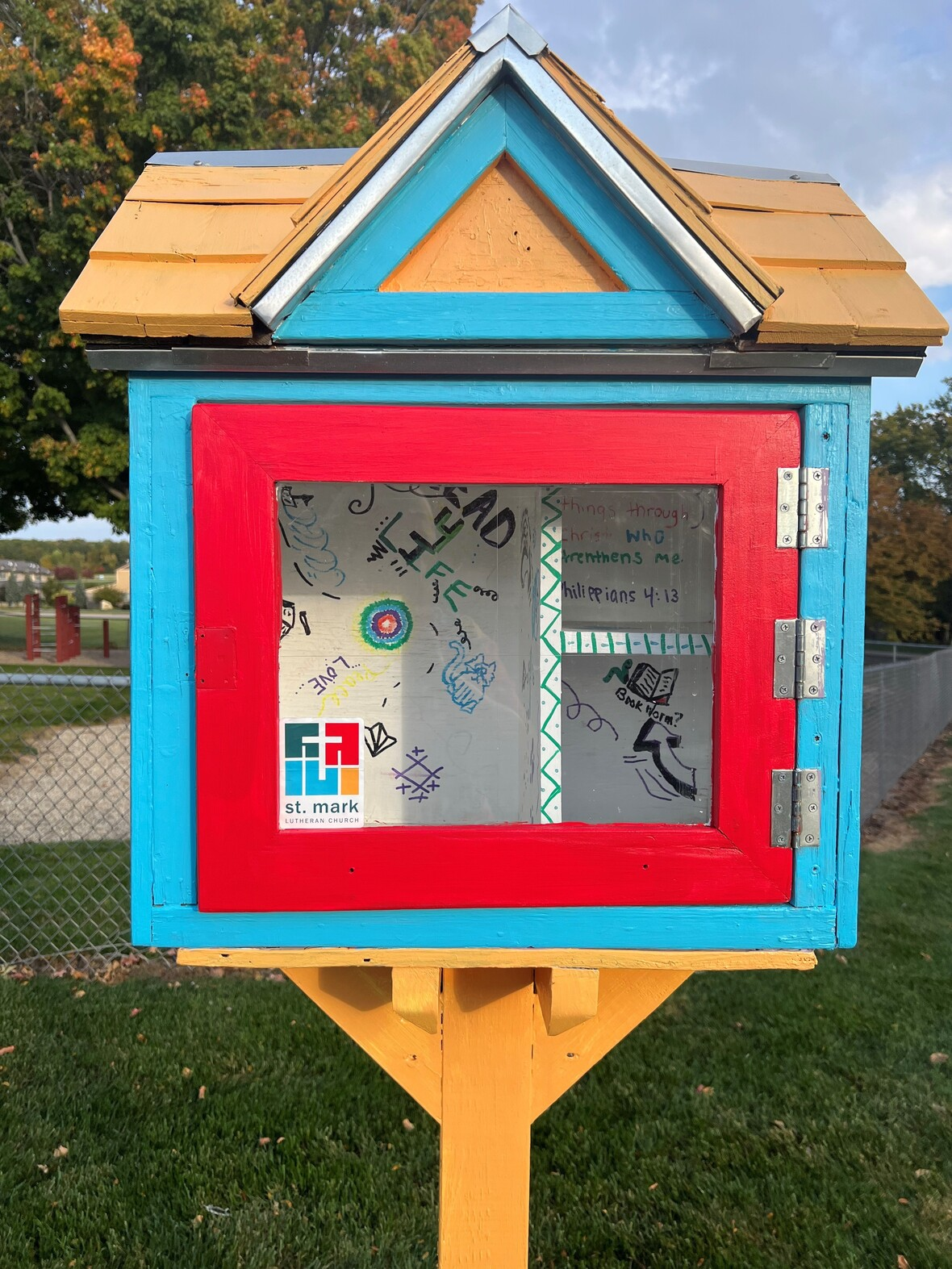table div table+table div table{width:100%;padding:0}table div table+table div table img{width:96.23%;padding:0;float:none}table div table+table div table td{width:100%;padding:0 1.88% 18px}/* styles */## This Week at St. Mark May 28 - June 4, 2023

 table div table+table+table+table div table{width:100%;padding:0}table div table+table+table+table div table img{width:96.23%;padding:0;float:none}table div table+table+table+table div table td{width:100%;padding:0 1.88% 18px}/* styles */## Sunday, May 28 Pentecost Sunday

9:00 A.M. Worship with Live-Stream
10:00 A.M. Fellowship Hour with coffee and donuts
11:00 A.M. Worship in Fellowship Hall

Office Closed

## Tuesday, May 30

9:00 A.M. Staff Meeting
10:30 A.M. St. Mark Duplicate Bridge

## Wednesday, May 31

9:30 A.M. St. Mark Ringers

## Thursday, June 1

9:00 A.M. Cherry Valley Bridge Group
6:30 P.M. Emotions Anonymous
6:30 P.M. Blue Lights

## Friday, June 2

7:30 A.M. St. Mark Breakfast Group at Denny's Restaurant

## Sunday, June 4

9:00 A.M. Worship with Live-Stream
10:00 A.M. Fellowship Hour with coffee and donuts
10:00 A.M. VBS Volunteer Orientation
11:00 A.M. Outdoor Worship
12:00 P.M. Bring Your Own Picnic
Everyone is invited! Drinks, popsicles, and ice cream novelties are provided.

 table div table+table+table+table+table+table div table{width:100%;padding:0}table div table+table+table+table+table+table div table img{width:96.23%;padding:0;float:none}table div table+table+table+table+table+table div table td{width:100%;padding:0 1.88% 18px}/* styles */## News and Events

 table div table+table+table+table+table+table+table+table div table{width:100%;padding:0}table div table+table+table+table+table+table+table+table div table img{width:96.23%;padding:0;float:none}table div table+table+table+table+table+table+table+table div table td{width:100%;padding:0 1.88% 18px}/* styles *//* styles */ Pentecost Sunday, May 28 Pentecost derives its name from the Jewish festival celebrating the harvest and the giving of the law on Mount Sinai fifty days after Passover. Fifty days after Easter, we celebrate the Holy Spirit as God’s presence within and among us. In Acts the Spirit arrives in rushing wind and flame, bringing God’s presence to all people. Paul reminds us that though we each have different capacities, we are unified in the Spirit that equips us with these gifts. Jesus breathes the Holy Spirit on his disciples, empowering them to forgive sin. We celebrate that we too are given the breath of the Holy Spirit and sent out to proclaim God’s redeeming love to all the world. 9:00 a.m. - Traditional Worship in the Sanctuary- Prelude will start at 8:55. 10:10 a.m. - Fellowship Hour with coffee and donuts 11:00 a.m. - Worship in Fellowship Hall If you are unable to be here, click here to join us at 8:55 for our live-stream service.
 table div table+table+table+table+table+table+table+table+table+table div table{width:100%;padding:0}table div table+table+table+table+table+table+table+table+table+table div table img{width:96.23%;padding:0;float:none}table div table+table+table+table+table+table+table+table+table+table div table td{width:100%;padding:0 1.88% 18px}/* styles */Outdoor Worship is Returning!
Sunday, June 4 our 11:00 service will return to the east lawn for the summer months! We will continue to worship as we did last year. In case of inclement weather, the 11:00 service will be held in Fellowship Hall. We hope you join us for the sunny cheerful service. Pack a lunch and stick around after the service for a picnic on the lawn! Ice cold drinks, popsicles, and ice cream novelties will be provided.

 table div table+table+table+table+table+table+table+table+table+table+table+table+table div table{width:100%;padding:0}table div table+table+table+table+table+table+table+table+table+table+table+table+table div table img{width:96.23%;padding:0;float:none}table div table+table+table+table+table+table+table+table+table+table+table+table+table div table td{width:100%;padding:0 1.88% 18px}/* styles */Mark your calendars: VBS is June 19-23!
Every day, our heroes will answer the call and spring into action, learning from Bible Heroes in stories from the Old and New Testaments to work together to bring peace, build each other up, and share with the whole world the great story of God’s love.

We will experience these stories together through music, storytelling, arts and crafts, games, and science. Come be part of our ultimate Hero Team!

Register and Volunteer here! Registration is for kids who are completing PreK 4 – 5th grade.VBS Hero Helpers Still Needed!
VBS is fast approaching, and the hero team needs our help to be ready! Can you answer the call?

Volunteers Needed:
Adult group leaders are needed for VBS week, June 19-23. Get to know a small group of heroes, guide them through their daily rotation, and have a whole lot of fun along the way!

Also needed are:
• Helpers to decorate the sanctuary and set up the learning spaces on June 18 at 10:00.
• Helpers to put up activity tent on June 18 at 12:00.
• Helpers to take down the tent on June 23 or 24 (we’ll work out the timing with available volunteers).

Items Needed:
• Large Rubbermaid-style storage/tote bins to borrow – mark them with your name so they can be returned
• 2 each of: wiffle ball, soccer ball, baseball, football, basketball, volleyball, tennis ball – mark them with your name so they can be returned

Email julie@stmarklc.com or visit our VBS website to join the Hero Team!

 table div table+table+table+table+table+table+table+table+table+table+table+table+table+table+table+table div table{width:100%;padding:0}table div table+table+table+table+table+table+table+table+table+table+table+table+table+table+table+table div table img{width:96.23%;padding:0;float:none}table div table+table+table+table+table+table+table+table+table+table+table+table+table+table+table+table div table td{width:100%;padding:0 1.88% 18px}/* styles */Shared Youth Ministry Director
After months of conversation, prayerful discernment, and careful planning, I am excited to announce that we have begun the search for a full-time Shared Youth Ministry Director! Read more.

 table div table+table+table+table+table+table+table+table+table+table+table+table+table+table+table+table+table+table div table{width:100%;padding:0}table div table+table+table+table+table+table+table+table+table+table+table+table+table+table+table+table+table+table div table img{width:96.23%;padding:0;float:none}table div table+table+table+table+table+table+table+table+table+table+table+table+table+table+table+table+table+table div table td{width:100%;padding:0 1.88% 18px}/* styles */Pr. Viereck’s book:
Thanks to a generous donor, free copies of Pr. Wayne Viereck’s book, Christian Faith in the 21st Century are available upon request from the church office. To request a copy, please call the office or email receptionist@stmarklc.com. Limit: one per household.

 table div table+table+table+table+table+table+table+table+table+table+table+table+table+table+table+table+table+table+table+table div table{width:100%;padding:0}table div table+table+table+table+table+table+table+table+table+table+table+table+table+table+table+table+table+table+table+table div table img{width:96.23%;padding:0;float:none}table div table+table+table+table+table+table+table+table+table+table+table+table+table+table+table+table+table+table+table+table div table td{width:100%;padding:0 1.88% 18px}/* styles */St. Mark "makers" will be crafting again on Monday, June 5, 2:00-4:00P.M. We meet in the St. Mark Cafe. Join us as a guest artist will lead us thru watercolor bead painting. Please rsvp to Melinda Alekna. Cost will be \$5 for supplies(bring water colors if you have them) Melinda.alekna@icloud.com or 815-871-0390. Come for the craft, conversation, and even a snack!

 table div table+table+table+table+table+table+table+table+table+table+table+table+table+table+table+table+table+table+table+table+table+table div table{width:100%;padding:0}table div table+table+table+table+table+table+table+table+table+table+table+table+table+table+table+table+table+table+table+table+table+table div table img{width:96.23%;padding:0;float:none}table div table+table+table+table+table+table+table+table+table+table+table+table+table+table+table+table+table+table+table+table+table+table div table td{width:100%;padding:0 1.88% 18px}/* styles */Do you have a few gently used books at home? We are looking for a few gently used books for the St. Mark Little Library, which is located by the playground. If you have a few books to donate, please put the books directly in the Little Library. We are not collecting the books inside the church. If the Little Library is full, please check the next time you are at St. Mark to see if there is room. We greatly appreciate your help in keeping books in the St. Mark Little Library.

 table div table+table+table+table+table+table+table+table+table+table+table+table+table+table+table+table+table+table+table+table+table+table+table+table div table{width:100%;padding:0}table div table+table+table+table+table+table+table+table+table+table+table+table+table+table+table+table+table+table+table+table+table+table+table+table div table img{width:96.23%;padding:0;float:none}table div table+table+table+table+table+table+table+table+table+table+table+table+table+table+table+table+table+table+table+table+table+table+table+table div table td{width:100%;padding:0 1.88% 18px}/* styles */Do you have the Church Directory App?
It's free and simple! It only takes a few minutes to download the app on your phone or create an online account. The Instant Church Directory App will always have updated members' information at your fingertips.

Mobiel App Login Instructions for Android Devices

For those of you who already have the app and you want to submit a new photo or if your contact information has changed, you can submit these changes from the app. Select Menu and Edit My Family. If you have any questions, please contact Jill Davenport at 815-398-3557 or jill@stmarklc.com.

 table div table+table+table+table+table+table+table+table+table+table+table+table+table+table+table+table+table+table+table+table+table+table+table+table+table+table div table{width:100%;padding:0}table div table+table+table+table+table+table+table+table+table+table+table+table+table+table+table+table+table+table+table+table+table+table+table+table+table+table div table img{width:96.23%;padding:0;float:none}table div table+table+table+table+table+table+table+table+table+table+table+table+table+table+table+table+table+table+table+table+table+table+table+table+table+table div table td{width:100%;padding:0 1.88% 18px}/* styles */table div table+table+table+table+table+table+table+table+table+table+table+table+table+table+table+table+table+table+table+table+table+table+table+table+table+table+table+table div table{width:100%;padding:0}table div table+table+table+table+table+table+table+table+table+table+table+table+table+table+table+table+table+table+table+table+table+table+table+table+table+table+table+table div table img{width:96.23%;padding:0;float:none}table div table+table+table+table+table+table+table+table+table+table+table+table+table+table+table+table+table+table+table+table+table+table+table+table+table+table+table+table div table td{width:100%;padding:0 1.88% 18px}/* styles */## Youth MinistryUPDATED Youth Group Schedule - Meets from 6:30-8:00 pm
May 28 - No Youth Group - Memorial Day Weekend
June 11 - 2nd Sunday Youth Group at Volcano Falls

In June Youth Group will be on Wednesday evenings. Please watch for more information regarding our Summer Youth Group events.

 table div table+table+table+table+table+table+table+table+table+table+table+table+table+table+table+table+table+table+table+table+table+table+table+table+table+table+table+table+table+table+table div table{width:100%;padding:0}table div table+table+table+table+table+table+table+table+table+table+table+table+table+table+table+table+table+table+table+table+table+table+table+table+table+table+table+table+table+table+table div table img{width:96.23%;padding:0;float:none}table div table+table+table+table+table+table+table+table+table+table+table+table+table+table+table+table+table+table+table+table+table+table+table+table+table+table+table+table+table+table+table div table td{width:100%;padding:0 1.88% 18px}/* styles */table div table+table+table+table+table+table+table+table+table+table+table+table+table+table+table+table+table+table+table+table+table+table+table+table+table+table+table+table+table+table+table+table+table div table{width:100%;padding:0}table div table+table+table+table+table+table+table+table+table+table+table+table+table+table+table+table+table+table+table+table+table+table+table+table+table+table+table+table+table+table+table+table+table div table img{width:96.23%;padding:0;float:none}table div table+table+table+table+table+table+table+table+table+table+table+table+table+table+table+table+table+table+table+table+table+table+table+table+table+table+table+table+table+table+table+table+table div table td{width:100%;padding:0 1.88% 18px}/* styles *//* styles */
 table div table+table+table+table+table+table+table+table+table+table+table+table+table+table+table+table+table+table+table+table+table+table+table+table+table+table+table+table+table+table+table+table+table+table+table+table div table{width:100%;padding:0}table div table+table+table+table+table+table+table+table+table+table+table+table+table+table+table+table+table+table+table+table+table+table+table+table+table+table+table+table+table+table+table+table+table+table+table+table div table img{width:96.23%;padding:0;float:none}table div table+table+table+table+table+table+table+table+table+table+table+table+table+table+table+table+table+table+table+table+table+table+table+table+table+table+table+table+table+table+table+table+table+table+table+table div table td{width:100%;padding:0 1.88% 18px}/* styles */## Remember In Our Prayers

 table div table+table+table+table+table+table+table+table+table+table+table+table+table+table+table+table+table+table+table+table+table+table+table+table+table+table+table+table+table+table+table+table+table+table+table+table+table+table div table{width:100%;padding:0}table div table+table+table+table+table+table+table+table+table+table+table+table+table+table+table+table+table+table+table+table+table+table+table+table+table+table+table+table+table+table+table+table+table+table+table+table+table+table div table img{width:96.23%;padding:0;float:none}table div table+table+table+table+table+table+table+table+table+table+table+table+table+table+table+table+table+table+table+table+table+table+table+table+table+table+table+table+table+table+table+table+table+table+table+table+table+table div table td{width:100%;padding:0 1.88% 18px}/* styles */## Our St. Mark Members:

Jeanne Zeschke, Ulla Kercher, Mark Honzel, Kris Steinke, Elton and Sandy Olson, Lorine Kortus, Beth Johnson, Carol Kilts, Fritzi Panjkovich, Jack Sowl, Jan Cotter, Diane Swanson, Sherri Lindquist, Bette Patterson.

## Family and Friends of St. Mark Members:

Suzanne Gyllin, Chris Nack, Ron Gentry, Terry Backen, John Ayotte, Carrie Kielty, Ed Sobczyk, Elizabeth Woodworth, Carrie Nelson, Bill Hart, Ellie Krepfle, Tom Montgomery, Jon Baylander, Jenny Hobby, Bill King, Carolyn King, Norma Comstock.

## Those serving in the military and their families:

Logan Gehrke, Hunter Haggestad, Tristan Davenport, Scot Hornick,
Bailey Panjkovich, Jim Tammen, Brandon Ballenger, Taylor Eversole.

Strengthen and encourage those in public health services and in the medical profession: care-givers, nurses, attendants, doctors, all who commit themselves to care for the sick and their families.

 table div table+table+table+table+table+table+table+table+table+table+table+table+table+table+table+table+table+table+table+table+table+table+table+table+table+table+table+table+table+table+table+table+table+table+table+table+table+table+table+table div table{width:100%;padding:0}table div table+table+table+table+table+table+table+table+table+table+table+table+table+table+table+table+table+table+table+table+table+table+table+table+table+table+table+table+table+table+table+table+table+table+table+table+table+table+table+table div table img{width:96.23%;padding:0;float:none}table div table+table+table+table+table+table+table+table+table+table+table+table+table+table+table+table+table+table+table+table+table+table+table+table+table+table+table+table+table+table+table+table+table+table+table+table+table+table+table+table div table td{width:100%;padding:0 1.88% 18px}/* styles *//* styles */ Summer Office Hours Monday through Thursday 9:00 A.M. - 3:00 P.M. Friday - 9:00 A.M. - 1:00 P.M. Telephone: 815-398-3557 Publications St. Mark Memos, the weekly e-newsletter, is distributed each Thursday. The deadline for submission is Monday by 3:00 P.M. Please click to send submissions. Worship Services Miss a Sunday at St. Mark? Please visit our website and watch the service online. Please click to watch.
 table div table+table+table+table+table+table+table+table+table+table+table+table+table+table+table+table+table+table+table+table+table+table+table+table+table+table+table+table+table+table+table+table+table+table+table+table+table+table+table+table+table+table div table{width:100%;padding:0}table div table+table+table+table+table+table+table+table+table+table+table+table+table+table+table+table+table+table+table+table+table+table+table+table+table+table+table+table+table+table+table+table+table+table+table+table+table+table+table+table+table+table div table img{width:96.23%;padding:0;float:none}table div table+table+table+table+table+table+table+table+table+table+table+table+table+table+table+table+table+table+table+table+table+table+table+table+table+table+table+table+table+table+table+table+table+table+table+table+table+table+table+table+table+table div table td{width:100%;padding:0 1.88% 18px}/* styles */